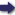## Korean independence outbreak beginning March 1st 1919

([S.l. :  s.n.,  1920?])

 Tools

## Search this bookPrev Page A18 Next``` A18 °" *^' Itl^j^tl ^?^S' the first article of which reads as follows•- of the 5ni?td sta?e?'^^''^'" T^'^^ and fpiend.^hip between the President Ehs excellent example aet by the Units'd .statoa of a recinrocallv friendly interest expressed in treaty was followed by Grelt Br?tlin lllt^.t'^TfTrf,^ treaty Signed at Hanyang on the 23th Kovembe? ' PartL^t^I f t^i?ri^^^?,^Sf oi^?^JI!:S CoL?fo^fn.^f^trif ^'^^ ^Sablf a^?an%^°At!"^' ^^^'^ "^ goof offices to br^^ioi? ^ tiartfs^who^^.f of'S?^""^^^ F^'^' *^° Chinese and Japanese plenipoten¬ tiaries who met at Shimonoseki to discuss terms of Sea-" ±i bPtw«^^ +>,<. orthe'fsrol'An^fl'lS^'^ l^' f"^"^"" °^ ^^^^^^^^^^^^^" dralt clause af follows?: *''" ■'^^^"""' plonipotentaries submitted a* ^"^ ^^HJ'^ ^^^^ suggestion Li Hung Chang wrote •- ness to reco,'n!zf Ih^u?? fS^ ^^.^^^^^s ago indicated its willing- the oomnll^e Seutrl^ft^ ii ?^ complete independence and fuarantee i^neeompiete neutrality of Korea and is read.y to Insert aurh i p+i^ ^io^d\ikewlt*r''*^'; ^r^ \^ "^^^ recipred^, Sucrs?ipu?ation '*■' 0?\oLrin^S?i:i!;n*^?iS*rJu^S^^%?^^^^^^rf^^^r^^L^r April? aalollo'wst?'^""' plenipotantary replied the next day, the 10th to al&lrf ?r?h?s"a??Slf a'"^;?^;:^?t"-^°"°^'^f^^ -=--^ plenipotentaries! ^^^"■^'^ ''''' vi^^.r.r.i..y prosontod by the Chinese mi and complete independent In* a. to^o^^^'^^I^^^L.^ir^^^I^^^if Lt: showef jI^rinMraSfn^'t^f ?f.r P^l''^>^od yost.rday Korea, in other words w^enrhtS - ^^^ --i^lepenaC^nce and sovereignty of iance to China Then in IR^? S" I country from its ancient alleg- o unmu. Then m 1882 we have the United States recognizin| the ```Prev Page A18 Next